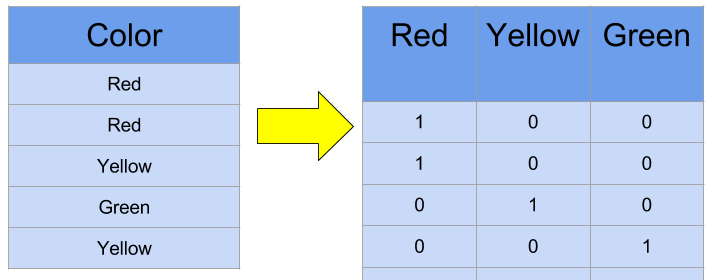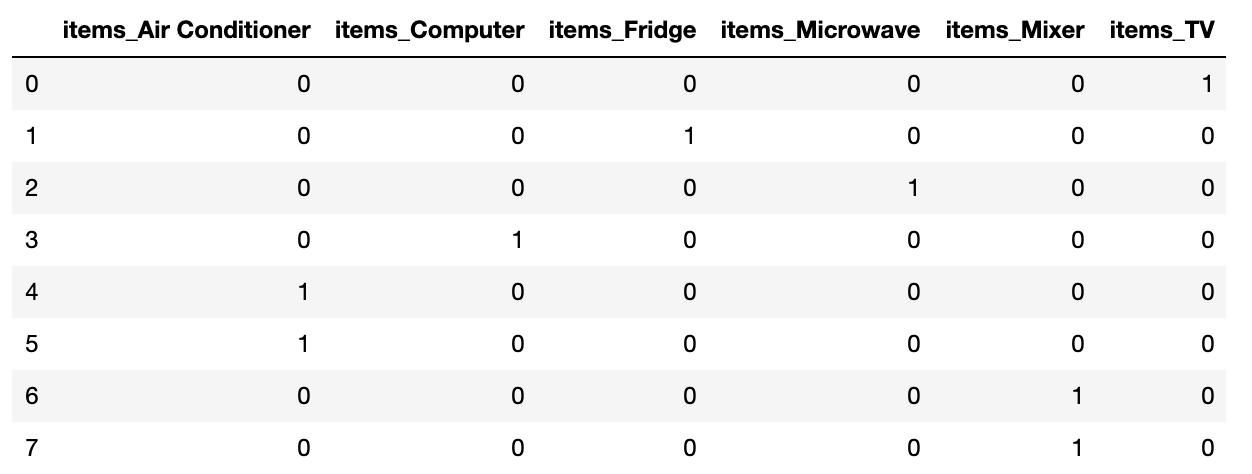# Data Preprocessing in Scikit-Learn

jiselectric·2021년 1월 6일
0

## Machine Learning

목록 보기
5/15Until now, we have learned about various machine learning techniques to enhance the performance of the model. However, handling and managing the data which the model processes are just as important as designing a sophisticated model.

First step in avoiding poor estimation starts from avoiding poor data (Garbage In, Garbage Out). Hence, we must carefully learn about data preprocessing.

Two Major Objectives in Data Preprocessing

• Remove NULL values
• Convert String Types to Num types

### Label Encoding

converting category features to num-values

from sklearn.preprocessing import LabelEncoder

items = ['TV', 'Fridge', 'Microwave', 'Computer', 'Air Conditioner',
'Air Conditioner', 'Mixer', 'Mixer']

# create LabelEncoder() object
encoder = LabelEncoder()

# use fit() & transform() to convert data
encoder.fit(items)
labels = encoder.transform(items)

print('Coverted Labels : ', labels)

Output

Coverted Labels :  [5 2 3 1 0 0 4 4]

Our label data in items object are not readable since they are composed of string values.

However, by creating LabelEncoder() object and processing the object through fit() & transform() method, we finally have converted label data.

print('Encoding Classes : ', encoder.classes_)

Output

Encoding Classes :  ['Air Conditioner' 'Computer' 'Fridge' 'Microwave' 'Mixer' 'TV']


We could check the classes of each converted label data.

print('Original Values Decoded : ', encoder.inverse_transform([5,2,3,1,0,0,4,4]))

Output

Original Values Decoded :  ['TV' 'Fridge' 'Microwave' 'Computer' 'Air Conditioner' 'Air Conditioner'
'Mixer' 'Mixer']


However, the problem of label encoding exists when applying specific ML-algorithms to the dataset. For particular algorithms, the model may grant emphasis on label data with higher numerical value resulting in data distortion.

### One-Hot Encoding

One-Hot Encoding grants the value of 1 to the unique value of each columns to prevent the issue of label encoding.Before starting the One-hot Encoding, we must keep in mind that OneHotEncoder() only accepts ..

• numerical label data
• 2-dimension array
from sklearn.preprocessing import LabelEncoder, OneHotEncoder
import numpy as np

items = ['TV', 'Fridge', 'Microwave', 'Computer', 'Air Conditioner',
'Air Conditioner', 'Mixer', 'Mixer']

# Crate LabelEncoding() Object
encoding = LabelEncoder()

# fit() & transform()
encoding.fit(items)
labels = encoding.transform(items)

# convert to 2-d array
labels = labels.reshape(-1, 1)

# Create OneHotEncoder() Object
oh_encoder = OneHotEncoder()

# fit() & transform()
oh_encoder.fit(labels)
oh_labels = oh_encoder.transform(labels)

print('One Hot Encoding Data')
print(oh_labels.toarray())
print('One Hot Encoding Data Shape')
print(oh_labels.shape)

Output

One Hot Encoding Data
[[0. 0. 0. 0. 0. 1.]
[0. 0. 1. 0. 0. 0.]
[0. 0. 0. 1. 0. 0.]
[0. 1. 0. 0. 0. 0.]
[1. 0. 0. 0. 0. 0.]
[1. 0. 0. 0. 0. 0.]
[0. 0. 0. 0. 1. 0.]
[0. 0. 0. 0. 1. 0.]]
One Hot Encoding Data Shape
(8, 6)


### Pandas : get_dummies()

Pandas provide get_dummies() API to easily support One-Hot Encoding. The API automatically converts string category to numerical values.

# get_dummies API
import pandas as pd

df = pd.DataFrame({'items' : ['TV', 'Fridge', 'Microwave', 'Computer', 'Air Conditioner',
'Air Conditioner', 'Mixer', 'Mixer']})

pd.get_dummies(df)

Output